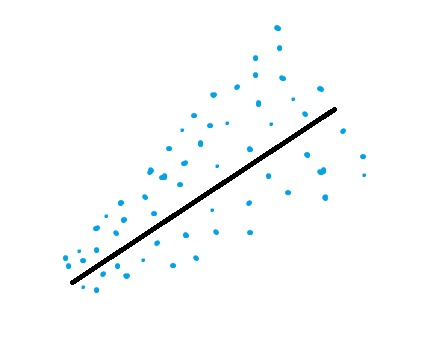# Breusch-Pagan-Godfrey Test: Definition

Regression Analysis > Breusch-Pagan-Godfrey Test

## What is the Breusch-Pagan-Godfrey Test?Heteroscedastic data.The Breusch-Pagan-Godfrey Test (sometimes shorted to the Breusch-Pagan test) is a test for heteroscedasticity of errors in regression. Heteroscedasticity means “differently scattered”; this is opposite to homoscedastic, which means “same scatter.” Homoscedasticity in regression is an important assumption; if the assumption is violated, you won’t be able to use regression analysis.

## Running the Test

The test statistic for the Breusch-Pagan-Godfrey test is:
N * R2 (with k degrees of freedom)
Where:

The test statistic approximately follows a chi-square distribution.

A small chi-square value (along with an associated small p-value) indicates the null hypothesis is true (i.e. that the variances are all equal).

Note that the Breush-Pagan test measures how errors increase across the explanatory variable, Y. The test assumes the error variances are due to a linear function of one or more explanatory variables in the model. That means heteroskedasticity could still be present in your regression model, but those errors (if present) are not correlated with the Y-values.

If you suspect that a small subset of values is causing heteroskedasticity, you can run a modified Breusch-Pagan test on those values alone.

Caution: Some authors (including Bickel(1978) and Koenker(1981) have suggested the Breusch-Pagan-Godfrey test statistic may not be accurate for non-normal data.

References:
Bickel, P.J., 1978, Using residuals robustly I: Test for heteroscedasticity and nonlinearity, Annals of Statistics 6, 266-291.
Breusch, T.S. and A.R. Pagan, 1979, A simple test for heteroscedasticity and random coefficient variation, Econometrica 47, 1287- 1294.
Koenker, R., 1981, A note on studentizing a test for heteroscedasticity, Journal of Econometrics 17, 107-l 12.

CITE THIS AS:
Stephanie Glen. "Breusch-Pagan-Godfrey Test: Definition" From StatisticsHowTo.com: Elementary Statistics for the rest of us! https://www.statisticshowto.com/breusch-pagan-godfrey-test/